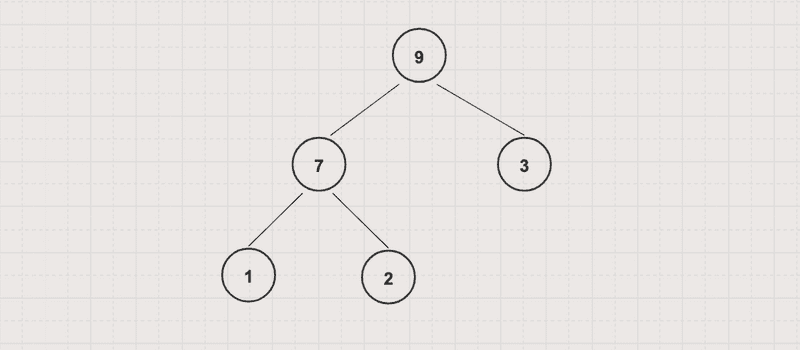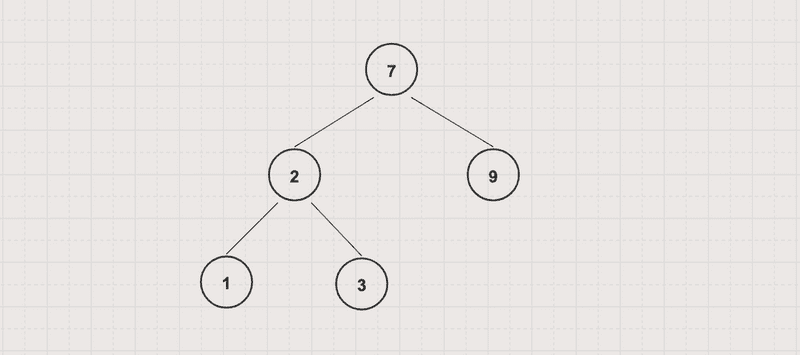# HelloKoding

Practical coding guides

# Binary Search Tree

Tree is a non-linear data structure consisting of a collection of nodes which are organized in hierarchy way

Binary Tree is a tree data structure in which each node has at most 2 children (left child and right child)Binary Search Tree, aka ordered/sorted binary tree, is a binary tree in which all parent node’s value are greater than theirs left child’s values and less than theirs right child’s values## Basic operations

• `traversal`, visits each node in a tree exactly once
• `insert`, adds a new node into a tree
• `delete`, removes a node from a tree

## Implementations

You can implement a binary search tree with either a linked list, static array (capacity restricted) or a dynamic array

The following give you a Binary Search Tree implementation example with Linked List

``````package com.hellokoding.datastructure;

public Node root;

private Node insert(Node node, int data) {
if (node == null) {
return new Node(data);
}

if (data < node.data) {
node.left = insert(node.left, data);
} else if (data > node.data)  {
node.right = insert(node.right, data);
}

return node;
}

public void insert(int data) {
this.root = insert(this.root, data);
}

public void inTraversal(Node node) {
if (node == null) return;

inTraversal(node.left);
System.out.println(node);
inTraversal(node.right);
}

public static void main(String[] args) {
tree.insert(7);
tree.insert(2);
tree.insert(3);
tree.insert(1);
tree.insert(9);

tree.inTraversal(tree.root);
}

public static class Node {
public int data;
public Node left;
public Node right;

public Node(int data) {
this.data = data;
}

@Override
public String toString() {
return String.format("data: %d, left: %d, right: %d", data, (left !=null ? left.data : null), (right != null? right.data : null));
}
}
}

``````